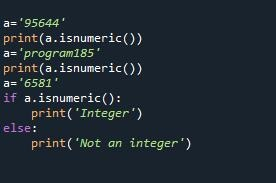# How to Check if String is Integer in Python

In python, if you call a numeric function on a non-numeric string, it may throw an exception. So it is advisable to check if string is integer in Python. There are several ways to do this. In this article, we will learn how to check if string is integer in Python.

## How to Check if String is Integer in Python

We will look at how to check if string is integer using try/except block, and using isdigit() function.

## 1. Using try…except

You can easily check if a string is an integer or not by calling int() function on it. If the string is not an integer it will throw an exception. To catch this exception, we will use a try-catch block.

```def is_int(s):
try:
int(s)
return True
except ValueError:
return False
```

In the above code, we simply call int() function on input string, from within a try…except block. If there is no exception, that is the input is an integer, it will return true, else it will return false.

Here is how you can call the above function.

```>>> print RepresentsInt("-123")
True
>>> print RepresentsInt("10.0")
False```

## 2. Using isdigit

You can also use isdigit() function to determine if a string is integer or not. Here is an example.

```>>> '36'.isdigit()
True```

But the isdigit() function works only with positive integers. If you need to check a negative number string, split the string into two parts – the minus sign and the number.

```>>> s = '-27'
>>> s.startswith('-') and s[1:].isdigit()
True```

In the above code, we call startswith() function just to check if it is negative, and then call isdigit() on the numerical part of string, starting from second character onwards. startswith() function returns true is the string starts with ‘-‘ sign. We combine this result with that of isdigit() function using AND operator.

Combining these both cases, you can create a function as shown below.

```def is_int(s):
if s in ('-', '+'):
return s[1:].isdigit()
return s.isdigit()```

In the above function, we first check if it the string’s first sign is + or -. If so, we call isdigit() function on the substring starting from second character of string. If there is no sign at the beginning, we directly call isdigit() function.

In this article, we will learn how to check if number is string in Python.